Скачать презентацию Dynamic Data Assimilation A historical view S Lakshmivarahan

f510f39e72c39525ea9ee0d402e3e121.ppt

• Количество слайдов: 23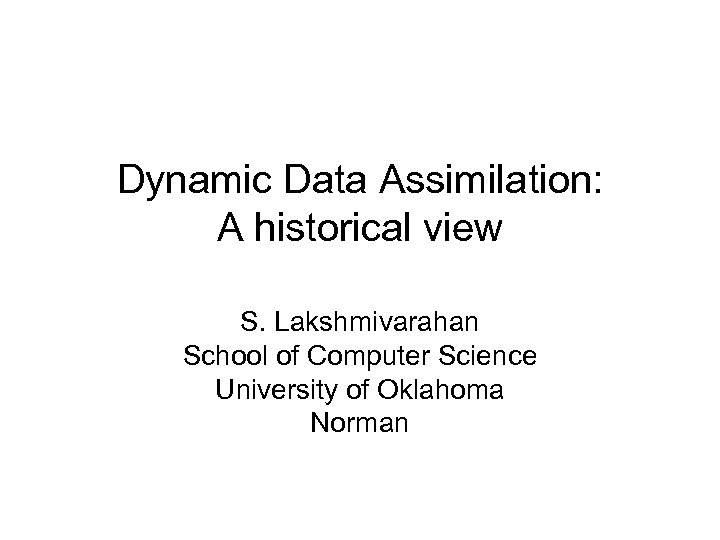Dynamic Data Assimilation: A historical view S. Lakshmivarahan School of Computer Science University of Oklahoma Norman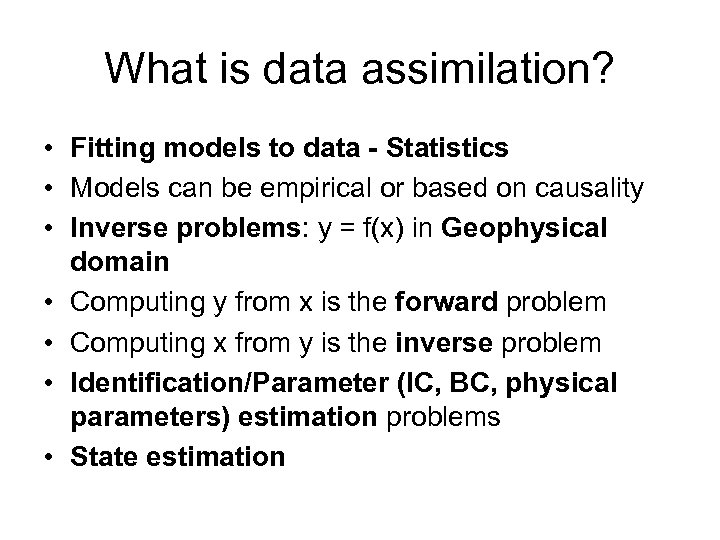What is data assimilation? • Fitting models to data - Statistics • Models can be empirical or based on causality • Inverse problems: y = f(x) in Geophysical domain • Computing y from x is the forward problem • Computing x from y is the inverse problem • Identification/Parameter (IC, BC, physical parameters) estimation problems • State estimationOther examples • Diagnosis of diseases from symptoms • CSI Miami • NTSB trying to pin down the causes for the collapse of the I-35 bridge in Minnesota or the TWA flight that crashed shortly after take-offWhy combine model and data? • Let x 1 and x 2 be two pieces of information about an unknown x with p 1 and p 2 be their variances • A linear estimate for x = a 1 x 1 + a 2 x 2 • Optimal (min. var) values for ai are: • a 1 = p 2 / (p 1 + p 2) and a 2 = p 1/ (p 1 + p 2) • Var(x) = p 1 p 2/(p 1 +p 2) < min (p 1, p 2)Dynamic model • • • Dynamic model: xk+1 = M[ xk ] + wk+1 Model space is Rn For simplicity consider discrete time - k M denotes the model – linear or nonlinear Randomness in models enters from two sources initial condition x 0 and forcing wk • wk denotes the model errors • This class of models are called Markov models in Rn • Analysis of continuous time needs a good working knowledge of stochastic calculus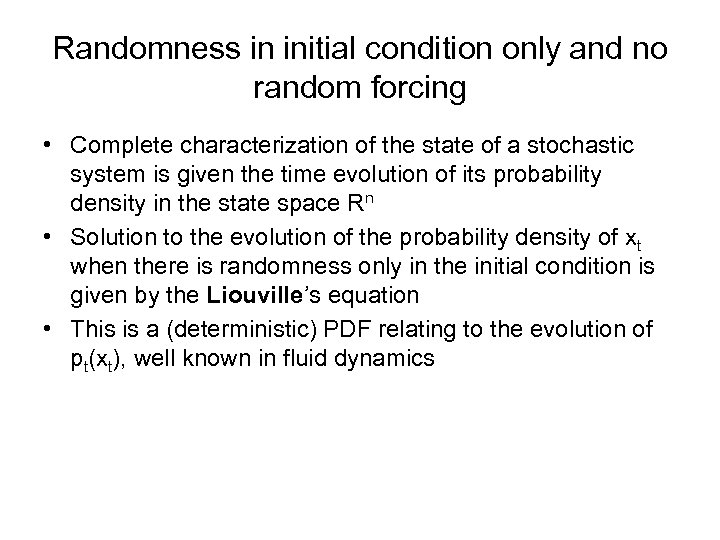Randomness in initial condition only and no random forcing • Complete characterization of the state of a stochastic system is given the time evolution of its probability density in the state space Rn • Solution to the evolution of the probability density of xt when there is randomness only in the initial condition is given by the Liouville’s equation • This is a (deterministic) PDF relating to the evolution of pt(xt), well known in fluid dynamics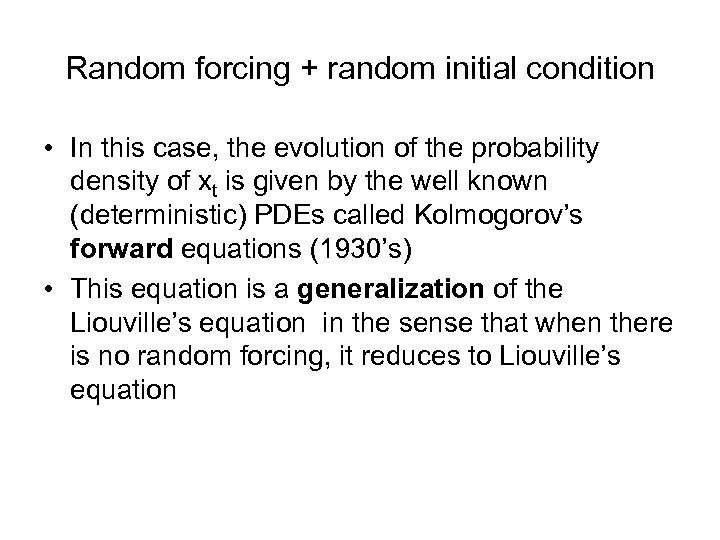Random forcing + random initial condition • In this case, the evolution of the probability density of xt is given by the well known (deterministic) PDEs called Kolmogorov’s forward equations (1930’s) • This equation is a generalization of the Liouville’s equation in the sense that when there is no random forcing, it reduces to Liouville’s equation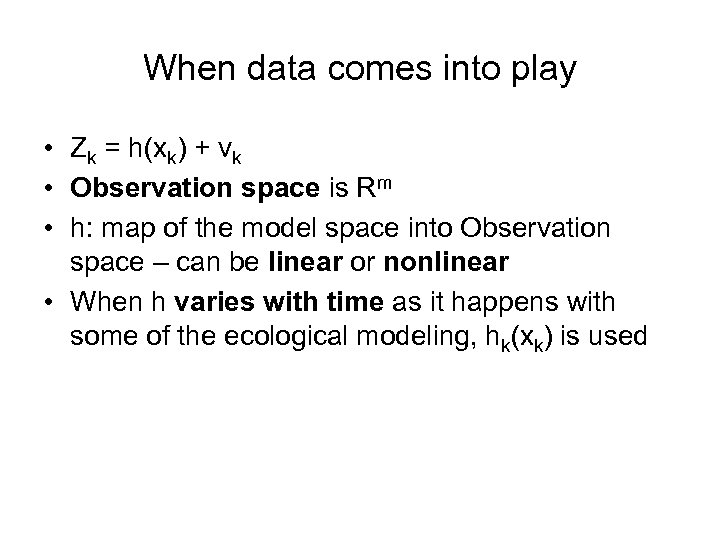When data comes into play • Zk = h(xk) + vk • Observation space is Rm • h: map of the model space into Observation space – can be linear or nonlinear • When h varies with time as it happens with some of the ecological modeling, hk(xk) is used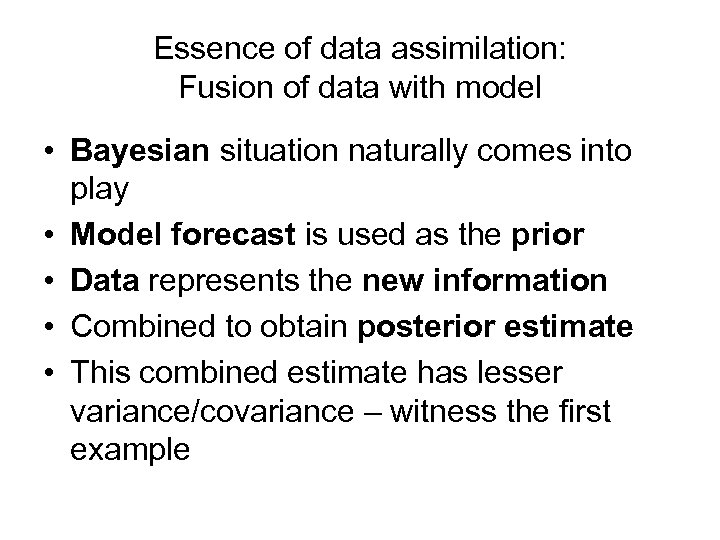Essence of data assimilation: Fusion of data with model • Bayesian situation naturally comes into play • Model forecast is used as the prior • Data represents the new information • Combined to obtain posterior estimate • This combined estimate has lesser variance/covariance – witness the first exampleWhere it all began? • Began with Gauss in 1801 with his discovery of the method of least squares • C. Gauss (1809) Theory of motion of heavenly bodies moving about the sun in conic sections, Dover edition published in 1963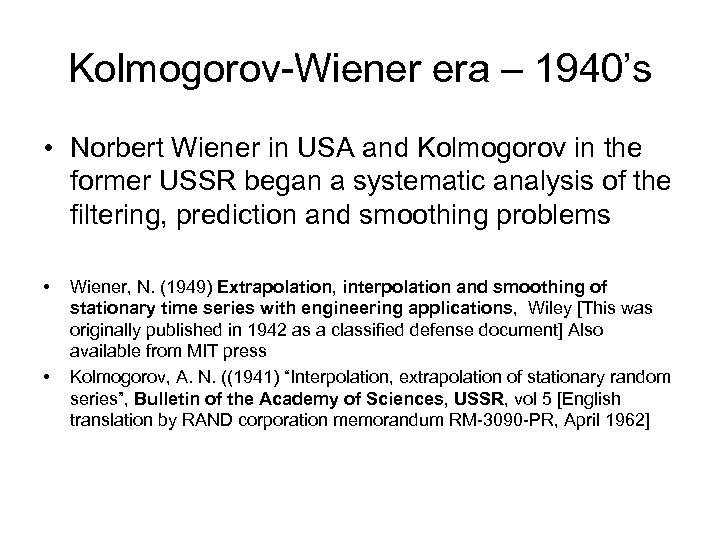Kolmogorov-Wiener era – 1940’s • Norbert Wiener in USA and Kolmogorov in the former USSR began a systematic analysis of the filtering, prediction and smoothing problems • • Wiener, N. (1949) Extrapolation, interpolation and smoothing of stationary time series with engineering applications, Wiley [This was originally published in 1942 as a classified defense document] Also available from MIT press Kolmogorov, A. N. ((1941) “Interpolation, extrapolation of stationary random series”, Bulletin of the Academy of Sciences, USSR, vol 5 [English translation by RAND corporation memorandum RM-3090 -PR, April 1962]The modern Kalman- Bucy Era – since 1960 • The previous methods for data assimilation were off-line techniques • Space travel presented much needed impetus to the development of on-line or sequential methods • • • Kalman, R. E. (1960) “ A new approach to linear filtering and prediction problems” ASME Transactions, Journal of Basic Engineering, Series D, vol 82, pp 35 -45 Kalman R. E. and R. S. Bucy ( 1961) “New results in linear filtering and prediction theory”, ibid, vol 83, pp 95 -108 These some of the most quoted papers in all of the literature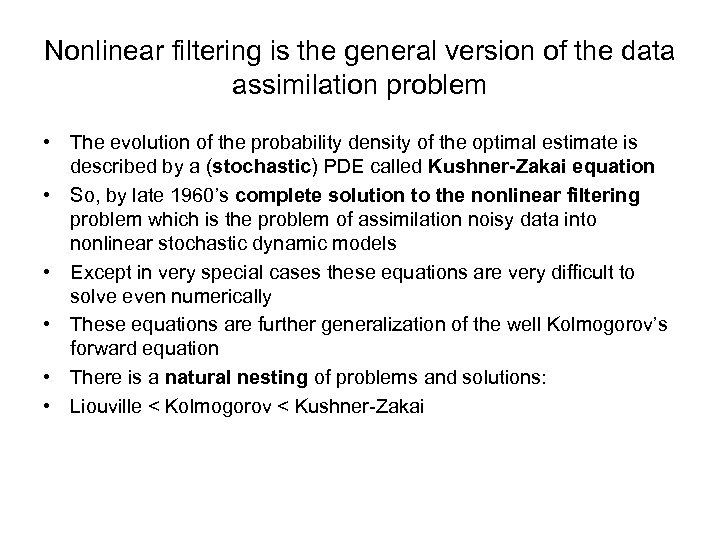Nonlinear filtering is the general version of the data assimilation problem • The evolution of the probability density of the optimal estimate is described by a (stochastic) PDE called Kushner-Zakai equation • So, by late 1960’s complete solution to the nonlinear filtering problem which is the problem of assimilation noisy data into nonlinear stochastic dynamic models • Except in very special cases these equations are very difficult to solve even numerically • These equations are further generalization of the well Kolmogorov’s forward equation • There is a natural nesting of problems and solutions: • Liouville < Kolmogorov < Kushner-Zakai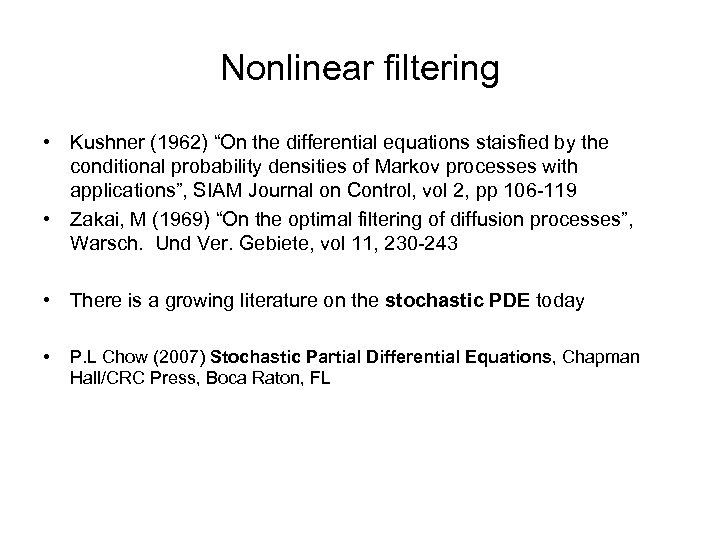Nonlinear filtering • Kushner (1962) “On the differential equations staisfied by the conditional probability densities of Markov processes with applications”, SIAM Journal on Control, vol 2, pp 106 -119 • Zakai, M (1969) “On the optimal filtering of diffusion processes”, Warsch. Und Ver. Gebiete, vol 11, 230 -243 • There is a growing literature on the stochastic PDE today • P. L Chow (2007) Stochastic Partial Differential Equations, Chapman Hall/CRC Press, Boca Raton, FLClassical approximations • • • Difficulty with solving Kushner-Zakai type equations forced us to look for easily computable moments of the state probability density, pt(xt) But again the moment closure problem forces us to settle for moment approximation First-order (extended Kalman) filter approximates the mean and covariance of xt using the first derivative term in the Taylor series of M(xt) around the known analysis Second-order filter – improved approximation using the first two derivatives in the Taylor series Since the 1960’s, these approximations have been applied to solve problems in Aerospace and Economics with considerable success Applications of Kalman filtering in Meteorology began only early 1980’sEnsemble Kalman filtering: an alternative to classical moment approximation • • • In meteorology, the problems sizes are rather large: n = a few tens of millions and m = a few millions Curse of dimensionality prevents application of the classical moment approximations Computation of the forecast and its covariance (resulting from n 3 complexity) is very time consuming To circumvent this difficulty, the notion of the (Monte Carlo type) ensemble filtering was introduced by G. Evensen (1994) in Meteorology There has been an explosion of literature on exsemble Kalman filtering in Meteorology G. Evensen (2007) Data Assimilation: The ensemble Kalman filter, Springer Verlag 279 pages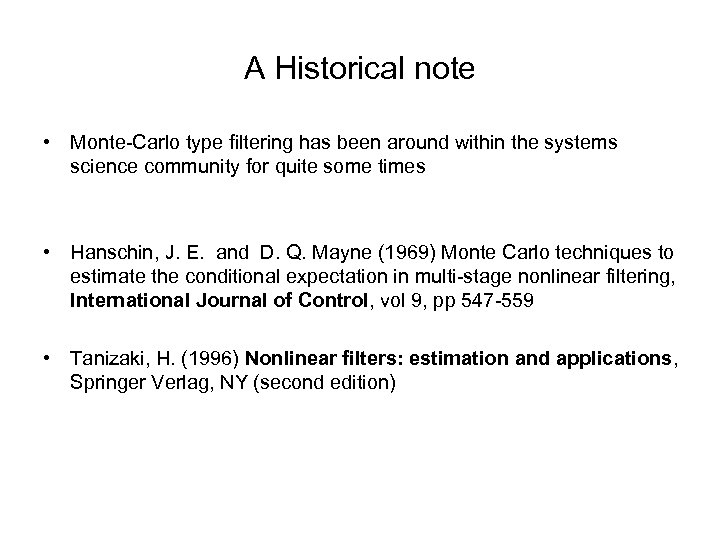A Historical note • Monte-Carlo type filtering has been around within the systems science community for quite some times • Hanschin, J. E. and D. Q. Mayne (1969) Monte Carlo techniques to estimate the conditional expectation in multi-stage nonlinear filtering, International Journal of Control, vol 9, pp 547 -559 • Tanizaki, H. (1996) Nonlinear filters: estimation and applications, Springer Verlag, NY (second edition)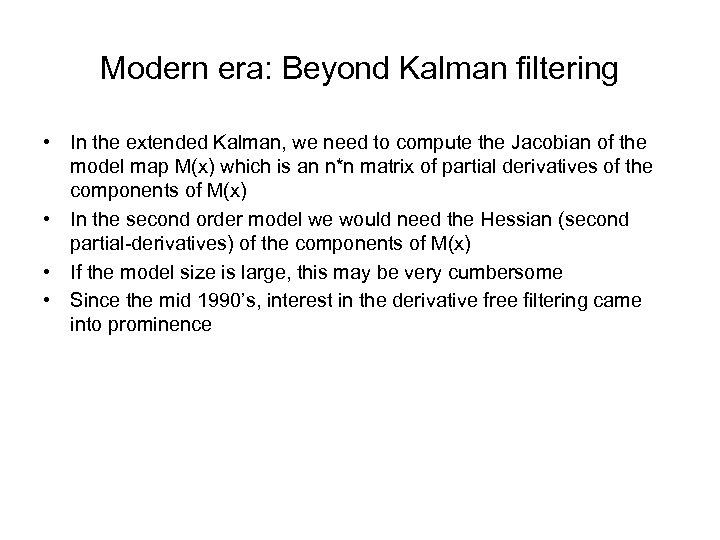Modern era: Beyond Kalman filtering • In the extended Kalman, we need to compute the Jacobian of the model map M(x) which is an n*n matrix of partial derivatives of the components of M(x) • In the second order model we would need the Hessian (second partial-derivatives) of the components of M(x) • If the model size is large, this may be very cumbersome • Since the mid 1990’s, interest in the derivative free filtering came into prominenceUnscented Kalman filtering • This is based on the use of deterministic ensemble – either a symmetric ensemble of size (2 n+1) or asymmetric ensemble of size (n+1) • Using this ensemble, moments of the model forecast can be computed up to third and/or fourth order accuracy • These are better than first and second order filters described earlier • This filters may be very useful for low dimensional ecological models • Julier, S. , J. Uhlmann and H. F. Durrant-Whyte (2002) A new emthod for the nonlinear trasformation of mean and covariance in filters and estimators, IEEE Transactions on Automatic Control, vol 45, 477 -482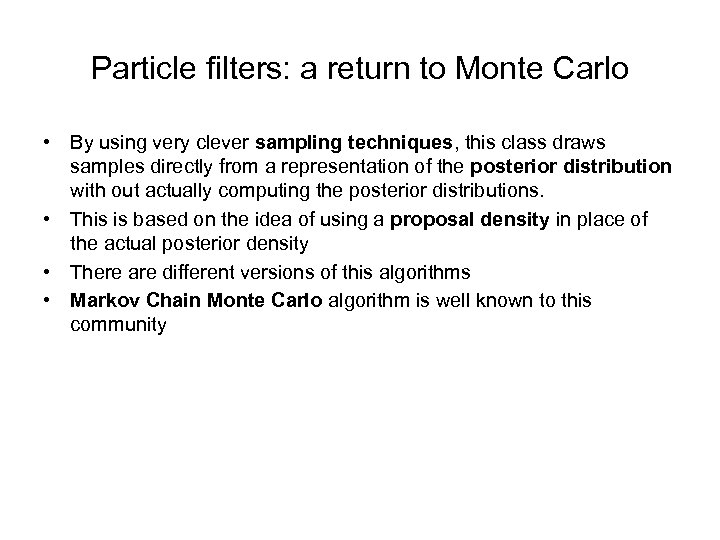Particle filters: a return to Monte Carlo • By using very clever sampling techniques, this class draws samples directly from a representation of the posterior distribution with out actually computing the posterior distributions. • This is based on the idea of using a proposal density in place of the actual posterior density • There are different versions of this algorithms • Markov Chain Monte Carlo algorithm is well known to this community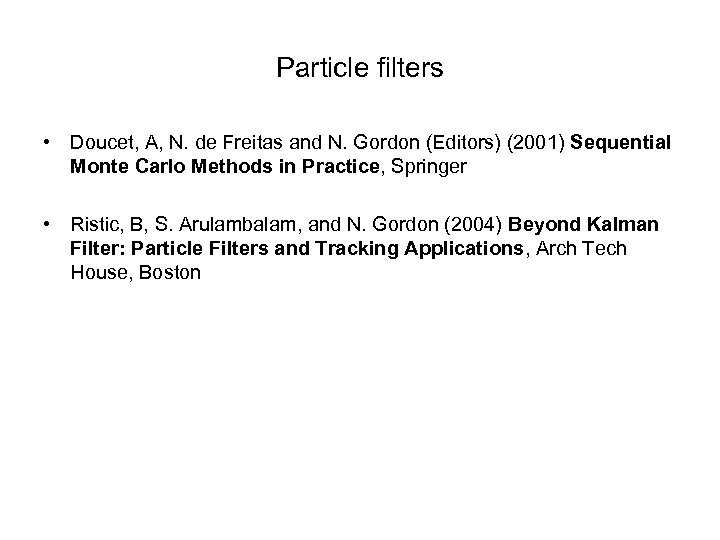Particle filters • Doucet, A, N. de Freitas and N. Gordon (Editors) (2001) Sequential Monte Carlo Methods in Practice, Springer • Ristic, B, S. Arulambalam, and N. Gordon (2004) Beyond Kalman Filter: Particle Filters and Tracking Applications, Arch Tech House, BostonReference • J. M. Lewis, S. Lakshmivarahan and S. K. Dhall (2006) Dynamic Data Assimilation, Cambridge University Press • This book grew out of teaching graduate level courses at OU and provides a comprehensive introduction to all aspects of Data assimilation • Classical retrieval algorithms • 3 D Var based on Bayesian frame work • 4 D Var – Adjoint methods • Linear and nonlinear filtering • Ensemble/ reduced rank filters • Predictability – deterministic and stochastic aspectsResearch and educational mission • Offer on-site intense short courses on specific aspects • Conduct hands on workshops • Work closely with researchers in this area in pushing the frontiers of the science